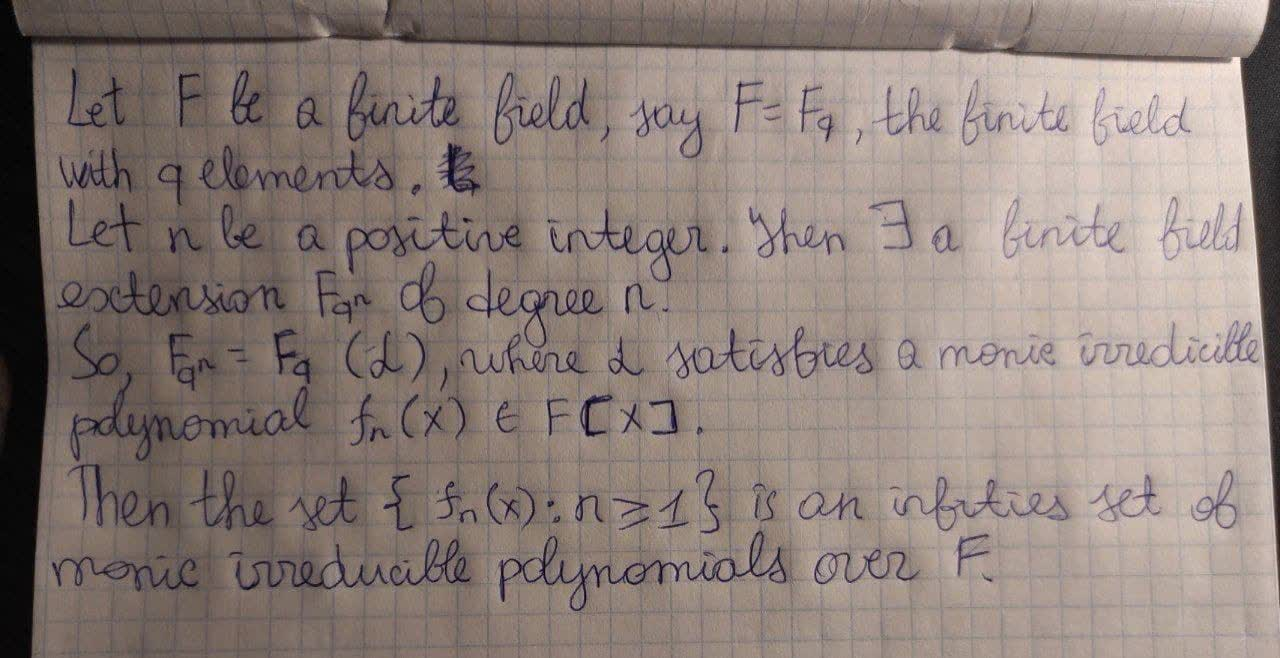# Let F be a field. Prove that there are infinitely many irreducible monic polynomialsQuestion
Abstract algebraLet F be a field. Prove that there are infinitely many irreducible monic polynomials2020-10-24
To prove the existence of infinitely many monic irreducible polynomials over any given field F.
A polynomial f(x) in F[x] is irreducible if f(x) cannot be factorized as f(x)=g(x)h(x), with g,h in F[x], ie coefficients in F. and deg g and h both greater than 1. In other words, f(x) is irreducbile if it does not be written as a non-trivial product.
If F is an infinite field, there is really nothing to prove: given any $$\displaystyle{a}\in{F}$$, x-a is monic and irreducible, as its degree is 1. The set $$\displaystyle{\left\lbrace{x}-{a},{a}\in{F}\right\rbrace}$$ is therefore an infinite set of monic irreducible polynomials
So the problem is mainly for the case when F is a finite field. In this case, the argument is as shown.
Let F be a finite field, say $$\displaystyle{F}={F}_{{q}}$$, the finite field with q elements.
Let n be a positive integer.
Then $$\displaystyle\exists$$ a finite field extension $$\displaystyle{F}_{{{q}^{{n}}}}$$ of degree n.
So, $$\displaystyle{F}_{{{q}^{{n}}}}={F}_{{q}}{\left(\alpha\right)}$$, where $$\displaystyle\alpha$$ satisfies a monic irreducible polynomial $$\displaystyle{{f}_{{n}}{\left({X}\right)}}\in{F}{\left[{x}\right]}.$$
Then the set $$\displaystyle{\left\lbrace{{f}_{{n}}{\left({x}\right)}}:{n}\ge{1}\right\rbrace}$$ is an infinite set of monic irreducile polynomials over F.### Relevant QuestionsLet F be a field, and $$\displaystyle{p}{\left({x}\right)}\in{F}{\left[{x}\right]}$$ an irreducible polynomial of degreed. Prove that every coset of $$\displaystyle{F}\frac{{{x}}}{{{p}}}$$ can be represented by unique polynomial of degree stroctly less than d. and moreover tha these are all distinct. Prove that if F has q elements, $$\displaystyle{F}\frac{{{x}}}{{{p}}}$$ has $$\displaystyle{q}^{{d}}$$ elements.Let F be i field with subfields K,L. Prove that there is a largest subfield of F contained in both K and L, and a smallest subfield of containung both K and L.Let RR sube K be a field extension of degree 2, and prove that K ~= CC. Prove that there is no field extension RR sube K of degree 3.Let F be a field and consider the ring of polynominals in two variables over F,F[x,y]. Prove that the functions sending a polyomial f(x,y) to its degree in x, its degree in y, and its total degree (i.e, the highest i+j where $$\displaystyle{x}^{{i}}{y}^{{i}}$$ appears with a nonzero coefficient) all fail o be norm making F[x,y] a Euclidean domain.Let a,b be coprime inegers. Prove that every integer x>ab-a-b can be written as na-mb where n,m where are non-negative inegers. Prove that ab-a-b connot be expressed ib this form.Let a,b be coprime integers. Prove that every integer x>ab-a-b can be written as na+mb where n,m are non negative integers. Prove that ab-a-b cannot be expressed in this form.Let $$\displaystyle{p},{q}\in\mathbb{Z}$$ be district primes. Prove that $$\displaystyle\mathbb{Q}{\left(\sqrt{{p}},\sqrt{{q}}\right)}=\mathbb{Q}{\left(\sqrt{{p}}+\sqrt{{q}}\right)}$$, and that $$\displaystyle\mathbb{Q}\subseteq\mathbb{Q}{\left(\sqrt{{p}}+\sqrt{{q}}\right)}$$ is a degree 4 extension.Let G be a group of order $$\displaystyle{p}^{{m}}$$ where p is prime number and m is a positive integer. Show that G contains an element of order p.